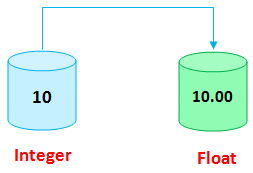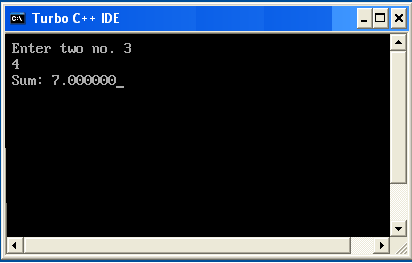# Type Casting in C

## Type Casting in C Programming

Type casting is process to convert a variable from one data type to another data type. For example if we want to store a integer value in a float variable then we need to typecast integer into float.## Example Type Casting in C

```#include <stdio.h>
#include <conio.h>

void main()
{
int a,b;
float sum;
clrscr();
printf("Enter two no. ");
scanf("%d%d",&a,&b);
sum=a+b;
printf("Sum: %f",sum);
getch();
}
```

Output